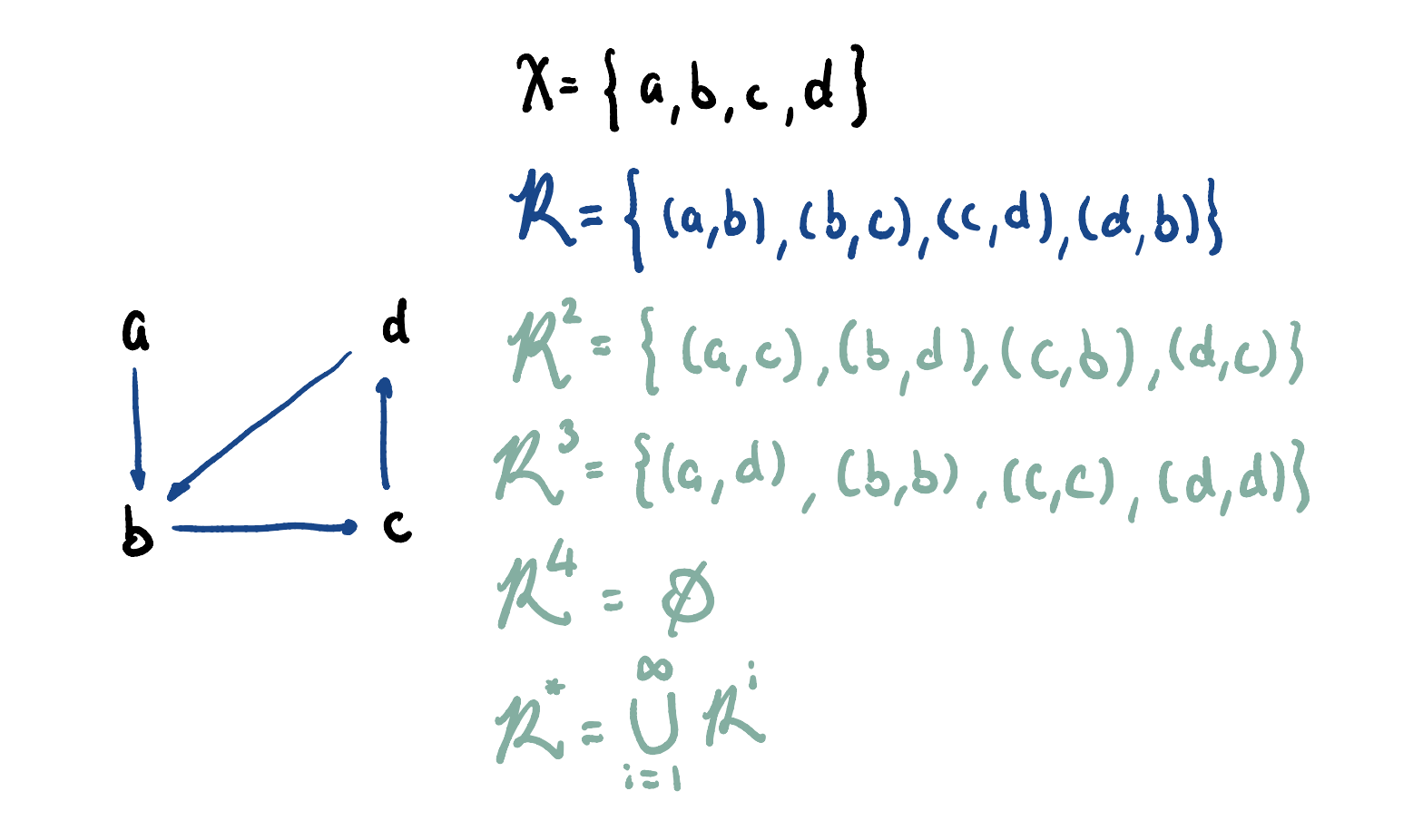Let:

• be the set of elements
• be the set of relations
• be the set of relations which can be reached from two hops of relations in
• For example, (a,c) is reached by going through (a,b) -> (b,c)
• is the entire transitive closure over